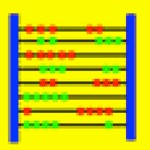# Abakus

Developed by K.-H. Erhard
USD 9.99

## App Details

Developer
K.-H. Erhard
Price
USD 9.99
Version
2.0
File Size
919.05 kB
Release Date
2011-02-14 19:27:08
Version Updated Date
2013-08-06 18:57:40
Release Notes
- Solution steps now available for all major functions
- Simplified user input since variables can be omitted in most cases
Languages
• English
compatibility

## App Description

Abakus is a computer algebra program which performs not only
arithmetic but even symbolic calculations of mathematical formulas.
Every calculation is performed directly on your smartphone or tablet,
i.e. an internet connection is not necessary.
Abakus shows detailed solution steps for all major functions.
This feature is unique and very useful for pupils and students
to prepare and check their math homework.

The current iOS version 2.0 contains the following functions:

Exact arithmetic calculations:
- Exact calculation of integer numbers of "arbitrary" size
- Calculation of real numbers with "arbitrary" precision
- Exact calculation of fractions (with solution steps)
- Least common multiple, greatest common divisor
- Factorial, binomial coefficient
- Prime numbers, prime factor separation, divisor set

Transforming mathematical formulas:
- Simplify, multiply, and factorize formulas; building the common denominator (with solution steps)
- Normalize, divide, and factorize polynomials; separate into partial fractions (with solution steps)

Discussing mathematical functions:
- Calculate function values
- Zeros, singularities, extremal points, turn points (with solution steps)
- Differentiation, integration (with solution steps)
- Calculation of limits (with solution steps)
- Graphical representation (2D and 3D)

Solving mathematical equations:
- Exact solution of linear and non-linear equations (with solution steps)
- Numerical solution of equations
- Exact solution of systems of linear equations (with solution steps)

Vector and matrix calculations:
- Simplify matrices (with solution steps)
- Invert matrices
- Calculate determinants
- Calculate Eigenvalues and Eigenvectors (with solution steps)

For example, the function "integrate + trace" applied to the formula
"x*sin(x)" with variable "x" yields the following output:

int(x*sin(x),x)
# Partial integration:
int(u(x)*v'(x),x) = u(x)*v(x)-int(u'(x)*v(x),x)
# u = x, v' = sin(x) => u' = 1, v = -cos(x)
-x*cos(x)-int(-cos(x),x)
# int(c*f(x),x) = c*int(f(x),x)
-x*cos(x)-(-1)*int(cos(x),x)
# Table
-x*cos(x)-(-1)*sin(x)
# Simplify
-x*cos(x)+sin(x).

Moreover Abakus contains a special editor for mathematical formulas.
So you can enter even complex expressions very fast.

At the moment, the following functions are supported:
sin,cos,tan,cot (trigonometric functions),
arcsin,arccos,arctan,arccot (inverse trigonometric functions),
sinh,cosh,tanh,coth (hyperbolic functions),
arsinh,arcosh,artanh,arcoth (inverse hyperbolic functions),
sqrt (square root),
crt (cubic root),
exp (exponential function),
ln (natural logarithm),
abs (Betrags function),
sgn (signum function).

Feasible operators are '+' (addition), '-' (subtraction),
'*' (multiplication), '/' (division), and '^' or '**' (power).

If you have a technical support question, please send an email to
abakusmath@t-online.de

Education

Education

Education